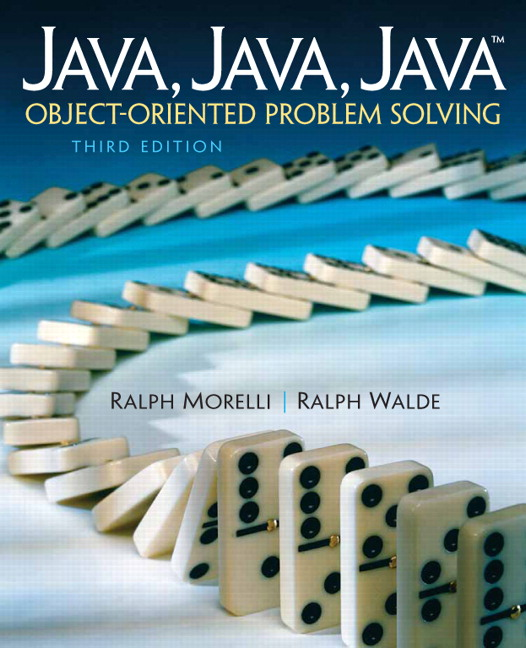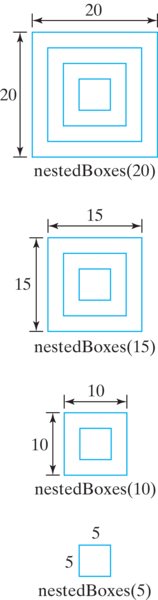# Java, Java, Java: Object-Oriented Problem Solving, 2022E

## Section12.2Recursive Definition

One place you might have already seen recursion is in mathematics. A recursive definition consists of two parts: a recursive part in which the nth value is defined in terms of the $$(n-1)$$st value, and a nonrecursive, boundary or base case, which defines a limiting condition.

### Subsection12.2.1Factorial: N!

For example, consider the problem of calculating the factorial of n—that is, n! for $$n \geq 0\text{.}$$ As you may recall, n! is calculated as follows:
n! = n * (n-1) * (n-2) * ... * 1, for n > 0

In addition, 0! is defined as 1. Let’s now look at some examples for different values of n:
4! = 4 * 3 * 2 * 1 = 24
3! = 3 * 2 * 1 = 6
2! = 2 * 1 = 2
1! = 1
0! = 1

As these examples suggest, n! can always be calculated in terms of $$(n-1)\text{!}$$ This relationship might be clearer if we rewrite the previous calculations as follows:
4! = 4 * 3 * 2 * 1 = 4 * 3! = 24
3! = 3 * 2 * 1     = 3 * 2! = 6
2! = 2 * 1         = 2 * 1! = 2
1!                 = 1 * 0! = 1
0!                 = 1

The only case in which we can’t calculate n! in terms of $$(n-1)\text{!}$$ is when n is 0. Otherwise, in each case we see that
n! = n * (n-1)!

This leads to the following recursive definition:
n! = 1          if n = 0    // Boundary (or base) case
n! = n * (n-1)! if n > 0    // Recursive case

Note that if we had omitted the base case, the recursion would have continued to $$(-1)\text{!}$$ and $$(-2)\text{!}$$ and so on.
The recursive case uses divide and conquer to break the problem into a smaller problem, but the smaller problem is just a smaller version of the original problem. This combination of self-similarity and divide and conquer is what characterizes recursion. The base case is used to stop or limit the recursion.

### Subsection12.2.2Drawing a Nested Pattern

As another example, consider the problem of drawing the nested boxes pattern in Figure 12.2.3.
The self-similarity occurs in the fact that for this pattern, its parts resemble the whole. The basic shape involved is a square, which is repeated over and over at an ever-smaller scale. A recursive definition for this pattern would be
Base case:      if side < 5 do nothing
Recursive case: if side >= 5
draw a square
decrease the side and draw a smaller
pattern inside the square

This definition uses the length of the square’s side to help define the pattern. If the length of the side is greater than or equal to 5, draw a square with dimensions $$side \times side\text{.}$$ Then decrease the length of the side and draw a smaller version of the pattern inside that square. In this case, the side variable will decrease at each level of the drawing. When the length of the side becomes less than 5, the recursion stops. Thus, the length of the side serves as the limit or bound for this algorithm.
You should note that the length of the side functions here like a parameter in a method definition: It provides essential information for the definition, just as a method parameter provides essential data to the method. Indeed, this is exactly the role that parameters play in recursive methods. They provide essential information that determines the method’s behavior.
Figure 12.2.4 illustrates how we would apply the definition. Suppose the side starts out at 20 and decreases by 5 at each level of recursion. Note that as you move from top to bottom across the four patterns, each pattern contains the one below it. A nestedBoxes(20) can be drawn by first drawing a $$20 \times 20$$ square and then drawing a nestedBoxes(15) pattern inside it. Similarly, a nestedBoxes(15) can be drawn by first drawing a $$15 \times 15$$ square and then drawing a nestedBoxes(10) pattern inside it. And so on.Figure 12.2.4. A trace of the nested boxes definition starting with a side of 20 and decreasing the side by 5 each time.
These examples illustrate the power of recursion as a problem-solving technique for situations that involve repetition. Like the iterative (looping) control structures we studied in Chapter 6, recursion is used to implement repetition within a bound. For recursive algorithms, the bound is defined by the base case, whereas for loops, the bound is defined by the loop’s entry condition. In either case, repetition stops when the bound is reached.

#### ExercisesSelf-Study Exercises

##### 1.Recursive definition: 2^n.
You can calculate $$2^n$$ by multiplying 2 by itself n times. For example, $$2^3$$ is $$2 \times 2 \times 2\text{.}$$ Note also that $$2^0=1\text{.}$$ Which of the following would be an appropriate recursive definition of $$2^n$$ for $$n \geq 0\text{?}$$
• 2^n =
0, if n = 0             // Base case
2 * 2^(n-1), if n > 0   // Recursive case

• The base case looks wrong.
• 2^n =
1, if n = 0             // Base case
n * 2^(n-1), if n > 0   // Recursive case

• It looks like this would result in $$n * n * ... * n\text{.}$$
• 2^n =
1, if n = 0             // Base case
2 * 2^(n-1), if n > 0   // Recursive case

• Right, this is a correct definition.
• None of the above
• Try again.
##### 2.Recursive definition: x^n.
Generalize your solution to the previous exercise by giving a recursive definition for $$x^n\text{,}$$ where $$x$$ and $$n$$ are both integers $$\geq 0\text{.}$$
• x^n =
1, if n = 0             // Base case
x * x^(n-1), if n > 0   // Recursive case

• Right, this is a correct definition.
• x^n =
1, if x = 0             // Base case
x * x^(n-1), if x > 0   // Recursive case

• The recursion should depend on $$n$$ not on $$x\text{.}$$
• x^n =
x, if n = 0             // Base case
x * x^(n-1), if n > 0   // Recursive case

• $$x^0 = 1\text{.}$$ In your definition $$x^0 = x\text{.}$$
• None of the above
• Try again.
##### 3.Equivalent definitions?
True or false, for $$side > 5\text{,}$$ the following recursive definitions for drawing nested boxes are equivalent.
Definition 1:
if side >= 5
draw a square
draw a smaller nested boxes inside the square

Definition 2:
Draw a square.
If side > 5
draw a smaller nested boxes inside the square

• True
• Both would produce the same result for $$side > 5\text{.}$$
• False
• Both would produce the same result for $$side > 5\text{.}$$